# Sequence pattern 1 - Consistent difference

The easiest pattern in a sequence to find is when there is a consistent difference between the terms, either addition or subtraction.

They also call this a linear sequence.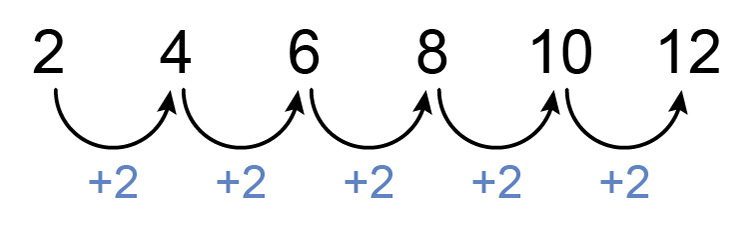This is one of the most famous linear sequences

Linear as in draw one line between each

and in this case, we get a difference of 2.

Example 1

Find the differences between each number below:

7,  11,  15  and  19

First, see if there is a consistent difference between each number.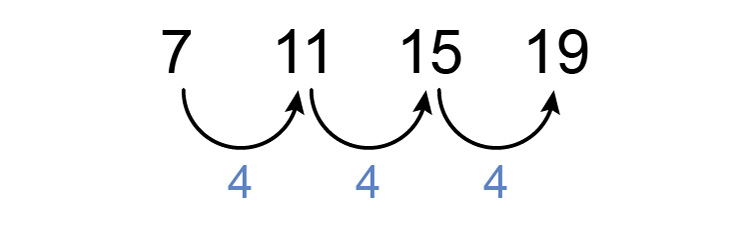Example 2

Find the difference between each number below:

0,  -3,  -6,  -9,  -12

First, see if there is a consistent difference between each term.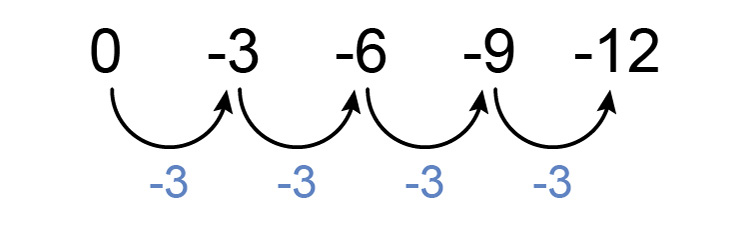Answer = -3

Example 3

Find the difference between

2,  9,  16,  23,  30

First, see if there is a consistent difference between each term.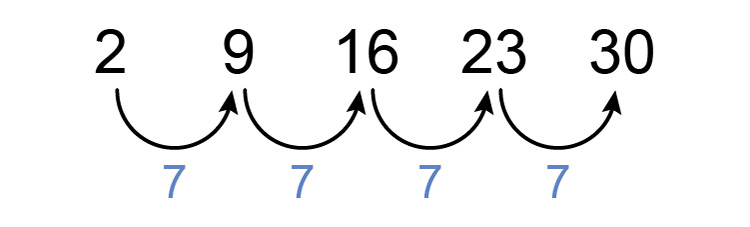Answer = 7

Example 4

Find the differences between each number below:

0,  -4,  -8,  -12,  -16

First, see if there is a consistent difference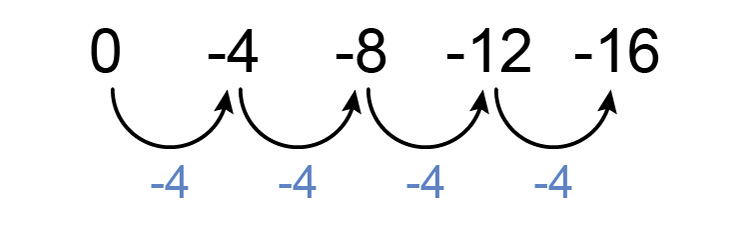Answer is -4

Example 5

Find the difference between each number below:

-1/2,  -1/4,  0,  1/4,  1/2

First, see if there is a consistent difference.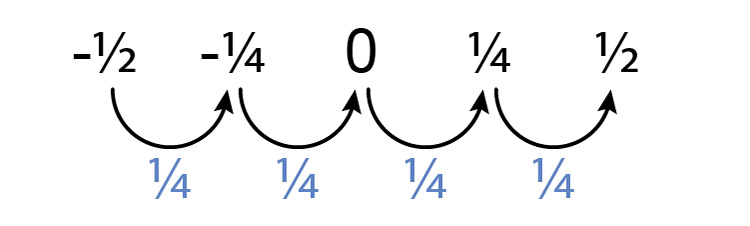Answer is 1/4

Example 6

Find the difference between each number below:

-1.1,  -1.5,  -1.9,  -2.3,  -2.7

First, see if there is a consistent difference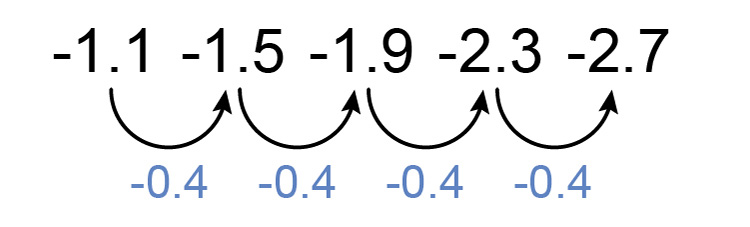Answer = -0.4